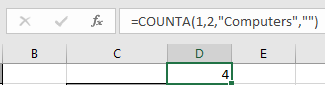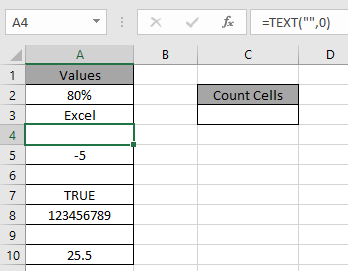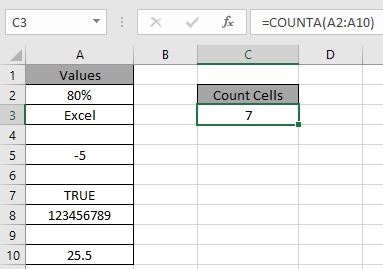# How to Use COUNTA Function in Excel

COUNTA function is a built in Excel function to calculate the Count of cells having Text, numbers or logic_test.
Syntax:

=COUNTA (value1, [value2], ...)
Note:
Non-Empty cells considered by COUNTA function

If we write a formula

=COUNTA(1,2,” Computers”,” ”)

It will return 4 as the count of cell having values are 4.
Here “ ” creates an empty cell but is counted by COUNTA function.Let’s understand more of this function using it an example.Here we have some values in column A including the formula in A4 cell.
Use the formula to count cells having any values.

=COUNTA(A2:A10)7 is the count of numeric value cells. Fully Empty cells are A6 & A9.
Use the COUNTA function to count text and logic_values in Excel.
Hope you understood how to use COUNTA function in Excel. Explore more articles on built-in Excel cell count function here. Please feel free to state your query or feedback for the above article.

Related Articles:

How to Use COUNT Function in Excel

Count Unique Values In Excel

Checking Whether Cells in a Range are Blank, and Counting the Blank Cells

How to Count Cells In A Range

How to Calculate Only If Cell is Not Blank in Excel

How to Use COUNT Function in Excel

How to Use COUNTA Function in Excel

The COUNTIFS Function in Excel

Popular Articles:

50 Excel Shortcuts to Increase Your Productivity

How to use the VLOOKUP Function in Excel

How to use the COUNTIF function in Excel 2016

How to Use SUMIF Function in Excel

Terms and Conditions of use

The applications/code on this site are distributed as is and without warranties or liability. In no event shall the owner of the copyrights, or the authors of the applications/code be liable for any loss of profit, any problems or any damage resulting from the use or evaluation of the applications/code.